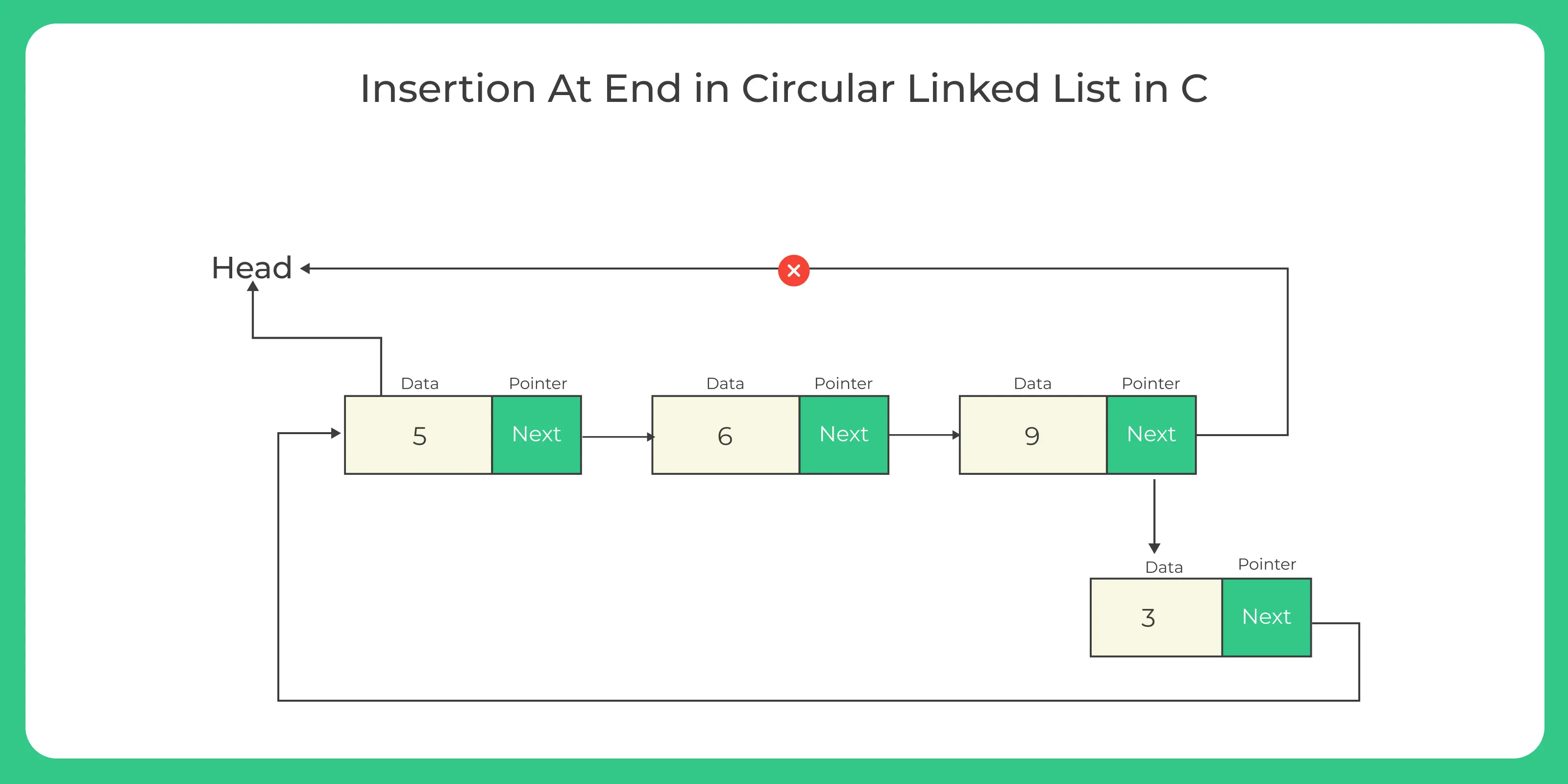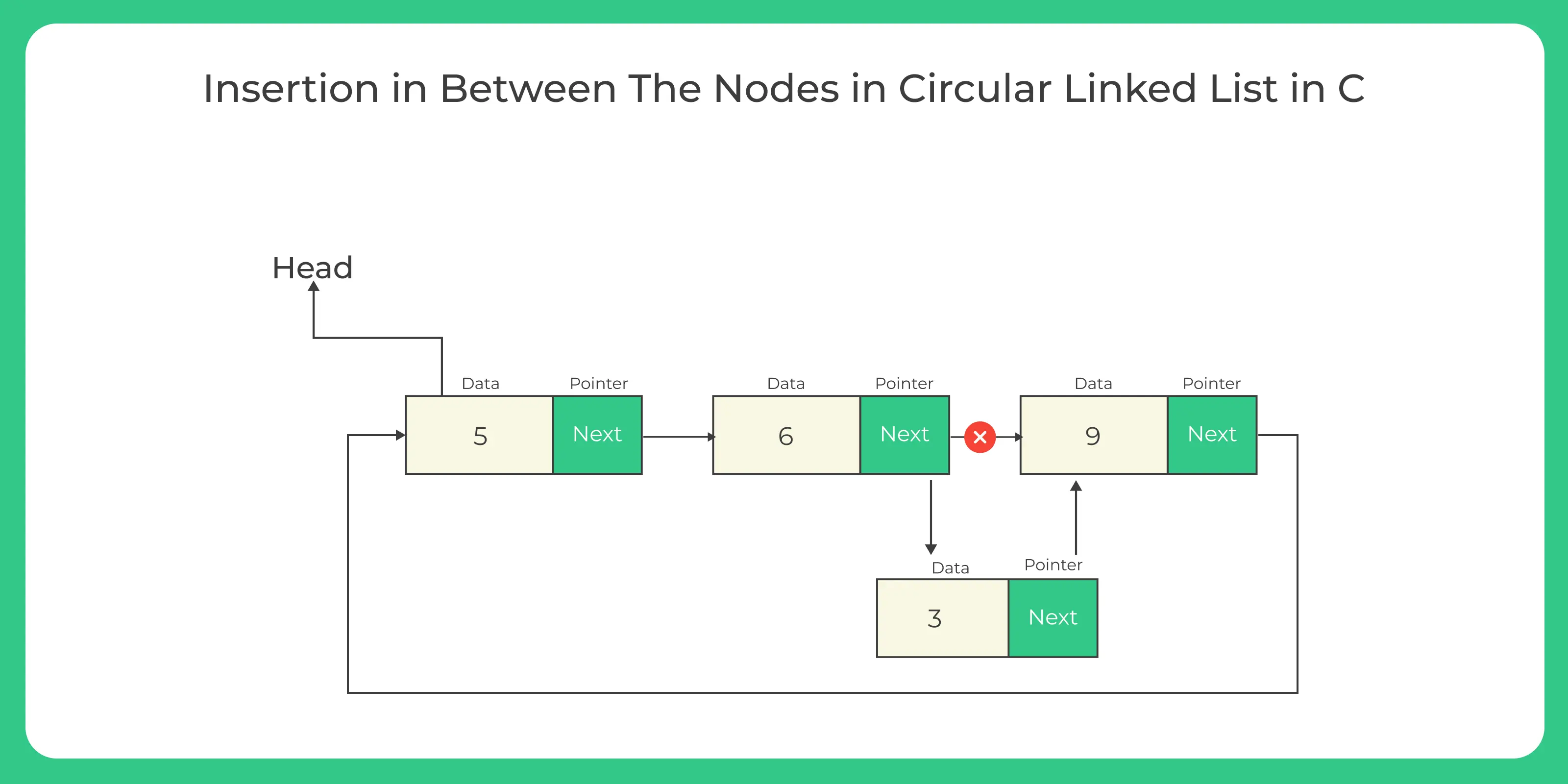# C Program for Insertion in circular linked list

## Learn how to insert node in the circular linked list

In the following section we will learn how to write a code in C Program for insertion in circular linked list, insertion means add a new node in the empty or already existed linked list.
We can perform insertion at different positions such as:-

• Insertion at the beginning
• Insertion at the end
• Insertion at the nth node## Types of insertion in circular linked list :-

1. Insertion at beginning:-

Insertion at starting in circular linked list in which we have to insert a new node before the head of the linked list, then the new node will become the head of the linked list.

2. Insertion at end:-

Insertion at last in circular linked list in which we have to add a new node at the end of the list, then the new node will become the tail of the linked list.

3. Insertion at nth node :-

Insertion at specific position means a new node is added according to the user wish in the linked list.

### Insertion at beginning in linked list the steps are followed :-

2. Then we have to take an extra pointer which points to the end node of the circular linked list.
3. Then we have a pointer that is pointing to the end node, then end node-> next will point to the first node.
4. At last follow the algorithm for insertion at beginning in circular linked list given below.

### Function for insertion at the beginning

```void insertStart (struct Node **head, int data)
{
struct Node *newNode = (struct Node *) malloc (sizeof (struct Node));
newNode->data = data;

// if its the first node being entered
{
return;
}

// if LL already as >=1 node

// traverse till last node in LL
{
curr = curr->next;
}
// assign LL's last node's next as this new node
curr->next = newNode;

// assign newNode's next as current head

// change head to this new node
}```

### Insertion at end in linked list the steps are followed :-

1. Make a new node.
2. Assign the new node next to circular list.
3. If the list is empty then return new node.
4. Assign the new node next to the front of the list.
5. Assign tail next to the new node.
6. Return the end node of the circular linked list.

### Function for insertion at the end

```void insertLast (struct Node **head, int data)
{
struct Node *newNode = (struct Node *) malloc (sizeof (struct Node));
newNode->data = data;

// if its the first node being entered
{
return;
}

// if LL already as >=1 node

// traverse till last node in LL
{
curr = curr->next;
}

// assign LL's current last node's next as this new node
curr->next = newNode;

// assign this new node's next as current head of LL
}```### Insertion in between the nodes in linked list the steps are followed :-

1. Make a new node and set the data.
2. Move to pos-1 position in the circular linked list.
3. Now link the next pointer of new node with the node pointed by the next pointer of current(pos-1) node.
4. After that join the next pointer of current node with the newly created node which means that the next pointer of current node will point to new node.
5. Now print the linked list.
6. Learn algorithm given below to understand better.

### Function for insertion at the specific position​

```void insertPosition (int data, int pos, struct Node **head)
//function to insert element at specific position
{
struct Node *newnode, *curNode;
int i;

{
printf ("List is empty");
}
if (pos == 1)
{
return;
}
else
{
newnode = (struct Node *) malloc (sizeof (struct Node));
newnode->data = data;
while (--pos > 1)
{
curNode = curNode->next;
}
newnode->next = curNode->next;
curNode->next = newnode;
}
}```### Code For Insertion In Circular Linked List

Run
```#include<stdio.h>
#include<stdlib.h>
struct Node
{
int data;
struct Node *next;
};
void insertStart (struct Node **head, int data)
{
struct Node *newNode = (struct Node *) malloc (sizeof (struct Node));
newNode->data = data;

// if its the first node being entered
{
return;
}

// if LL already as >=1 node

// traverse till last node in LL
{
curr = curr->next;
}
// assign LL's last node's next as this new node
curr->next = newNode;

// assign newNode's next as current head

// change head to this new node
}

void insertLast (struct Node **head, int data)
{
struct Node *newNode = (struct Node *) malloc (sizeof (struct Node));
newNode->data = data;

// if its the first node being entered
{
return;
}

// if LL already as >=1 node

// traverse till last node in LL
{
curr = curr->next;
}

// assign LL's current last node's next as this new node
curr->next = newNode;

// assign this new node's next as current head of LL
}

void insertPosition (int data, int pos, struct Node **head)
//function to insert element at specific position
{
struct Node *newnode, *curNode;
int i;

{
printf ("List is empty");
}
if (pos == 1)
{
return;
}
else
{
newnode = (struct Node *) malloc (sizeof (struct Node));
newnode->data = data;
while (--pos > 1)
{
curNode = curNode->next;
}
newnode->next = curNode->next;
curNode->next = newnode;
}
}

{
// if there are no node in LL
return;

//need to take care of circular structure of LL
do
{
printf ("%d ", temp->data);
temp = temp->next;

}
printf ("\n");
}

int main ()
{

printf("Insert at beginning: ");

printf("Insert at End: ");

printf("Insert at Specific Position: ");

return 0;
}
```

### Output

```Insert at beginning: 1 2
Insert at End: 1 2 30 40
Insert at Specific Position: 1 2 5 30 40
```

### Related Banners

Get PrepInsta Prime & get Access to all 200+ courses offered by PrepInsta in One Subscription

## Get over 200+ course One Subscription

Courses like AI/ML, Cloud Computing, Ethical Hacking, C, C++, Java, Python, DSA (All Languages), Competitive Coding (All Languages), TCS, Infosys, Wipro, Amazon, DBMS, SQL and others

## Checkout list of all the video courses in PrepInsta Prime Subscription

• Introduction to Circular Linked List
• Circular Linked List in –
C | C++ | Java
• Insertion in Circular Linked List –
C | C++ | Java
• Insertion at the beginning–
C | C++ | Java
• Insertion at the end –
C | C++ | Java
• Insertion at nth position –
C | C++ | Java
• Deletion in Circular Linked List –
C | C++ | Java
• Deletion from beginning in Circular Linked List –
C | C++ | Java
• Deletion from nth position in Circular Linked List –
• Deletion from end in Circular Linked List –
C | C++ | Java
• Insertion and Deletion in Circular Linked List – C | C++ | Java
• Split a Circular Linked List in two halves –
C | C++ | Java
• Count nodes in Circular Linked List –
C | C++ | Java
• Sorted Insert In Circular Linked List –
C | C++ | Java
• Insertion in the middle in Circular Linked List –
C | C++ | Java